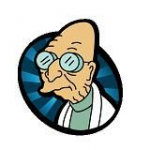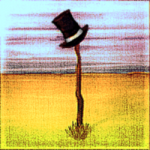###Author Topic: Page flipping animation  (Read 5813 times)

####Kitty Hello

• code monkey
• Prof. Inline
••• Posts: 10790
• here on my island the sea says 'hello'##### Page flipping animation
« on: 2010-Apr-06 »
Code: (glbasic) [Select]
`// --------------------------------- //// Project: PageFlip// Start: Tuesday, April 06, 2010// IDE Version: 7.308SYSTEMPOINTER TRUE FOR i=0 TO 999 DRAWRECT RND(500), RND(500), 32, 32, RGB(RND(255), RND(128), RND(255) ) NEXT GRABSPRITE 0, 0,0, 512, 512 DRAWRECT 0,0,600,600,RGB(255,255,255) FOR i=0 TO 999 PRINT "Page 2", RND(500), RND(500) NEXT GRABSPRITE 1, 0,0, 512, 512 // flip thingy WHILE TRUE LOCAL mx%, my%, b1%, b2% MOUSESTATE mx, my, b1, b2 DRAWRECT 0,0,777,777,RGB(128,128,128) FlipPageDraw(0, 50,50,480,320, mx, my, FALSE) SHOWSCREEN WEND//! Draws a Flip-Page animation frame// \param x%,y% - position of left/top page sheet// \param w%,h% - width/height of the paper sheet -> these coords are used as texture points, too -> texture can be bigger, but starts at 0,0!// \param px%,py% - point where you "drag" the bottom right corner toFUNCTION FlipPageDraw%: id%, x, y, w, h, px, py, bLeftToRight% // draw a frame for where the paper would be DRAWRECT x,y,w,h, RGB(0xaa, 0xaa, 0xaa) IF bLeftToRight% INC x, w w=-w ENDIF IF (w>0 AND px >= x+w) OR (w<0 AND px <=x+w)  // tear the paper to the right STARTPOLY id, 0 POLYVECTOR x,y, x,y,0xffffff POLYVECTOR x,y+h,x,y+h,0xffffff POLYVECTOR x+w,y+h,x+w,y+h,0xffffff POLYVECTOR x+w,y,x+w,y,0xffffff ENDPOLY RETURN ENDIF // Find the flip axis LOCAL fcx, fcy // flip center point. Center between bottom right corner and mouse pos (on bending line) fcx = (x+w+px)/2 fcy = (y+h+py)/2 // now get the direction of the flip edge (normal to the line px,py -> x+w,y+h)LOCAL nx, ny nx = y+h - py ny = -(x+w - px) // normalize LOCAL nl = SQR(nx*nx + ny*ny) IF nl < 1E-5 THEN RETURN // nothing visible nx = nx / nl ny = ny / nl LOCAL tx, ty // top point (corner of flipped paper) LOCAL t2x, t2y // 2nd top point (if the bending line hits the top edge, intersection of bottom paper top and bending line) LOCAL bx, by // bottom point // if nx=0 we get div/0 -> make a very small value IF ABS(nx)<=1E-5 THEN nx=1E-4 IF ABS(ny)<=1E-5 THEN ny=1E-4 // Get intersection y or bending line with the bottom paper edge u = (y+h-fcy)/ny bx = fcx+u*nx by = fcy+u*ny // top point, intersection with right edge // px + u*nx = x+w LOCAL u = (x+w-fcx)/nx ty = fcy + u*ny LOCAL ty_first = ty // py+u*ny = y u = (y-fcy)/ny // intersection of bending line with top edge t2x = fcx+u*nx t2y = y // find the real top point now // That's a bit tricky now // the folded edge is perpendicular to the bottom/mouse point line // _and_ the length equals the distance of the current top point to top/right corner LOCAL dx, dy // direction of bottom intersection point to mouse point dx = px-bx dy = py-by nl = SQR(dx*dx+dy*dy) dx=dx/nl dy=dy/nl // length of bended page at top edge u = x+w - t2x IF w<0 THEN u=-u // flip direction if turning page left to right tx = t2x+dx * u // move top bending intersection perpendicular to lower teared paper edge ty = t2y+dy * u // dont' fold paper too much off the right // OR  we're far too left with the mouse IF (w>0 AND tx > x+w) OR (w<0 AND tx < x+w) OR (py<y+h AND ty>y) tx = x+w ty = ty_first t2x=tx t2y=ty ENDIF // Draw the front of that page STARTPOLY id, 0 POLYVECTOR t2x,t2y,t2x,t2y,0xffffff POLYVECTOR bx, by, bx ,by ,0xffffff POLYVECTOR x, y+h, x  ,y+h,0xffffff POLYVECTOR x, y,   x  ,y  ,0xffffff POLYVECTOR tx, y,  tx ,y  ,0xffffff ENDPOLY // Draw the fine back of the page // b, p, t - triangle STARTPOLY -1, 0 POLYVECTOR tx, ty, 0,0,0xffffff POLYVECTOR t2x,t2y,0,0,0xcccccc POLYVECTOR bx, by, 0,0,0xcccccc POLYVECTOR px, py, 0,0,0xffffff ENDPOLYENDFUNCTION`
Still some work to do to make it look really well - but it's a start...

 Option to flip from left to right added.
« Last Edit: 2011-Jun-30 by Kitty Hello »

####thomasp

• Mc. Print
•• Posts: 14##### Re: Page flipping animation
« Reply #1 on: 2010-Apr-06 »
Schick!####bigsofty

• Community Developer
• Prof. Inline
••• Posts: 2721##### Re: Page flipping animation
« Reply #2 on: 2010-Apr-06 »
Very cool effect!
Cheers,

Ian.

“It is practically impossible to teach good programming style to students that have had prior exposure to BASIC.  As potential programmers, they are mentally mutilated beyond hope of regeneration.”
(E. W. Dijkstra)

####Hatonastick

• Dr. Type
••• Posts: 474Brilliant!  I love that effect mate.